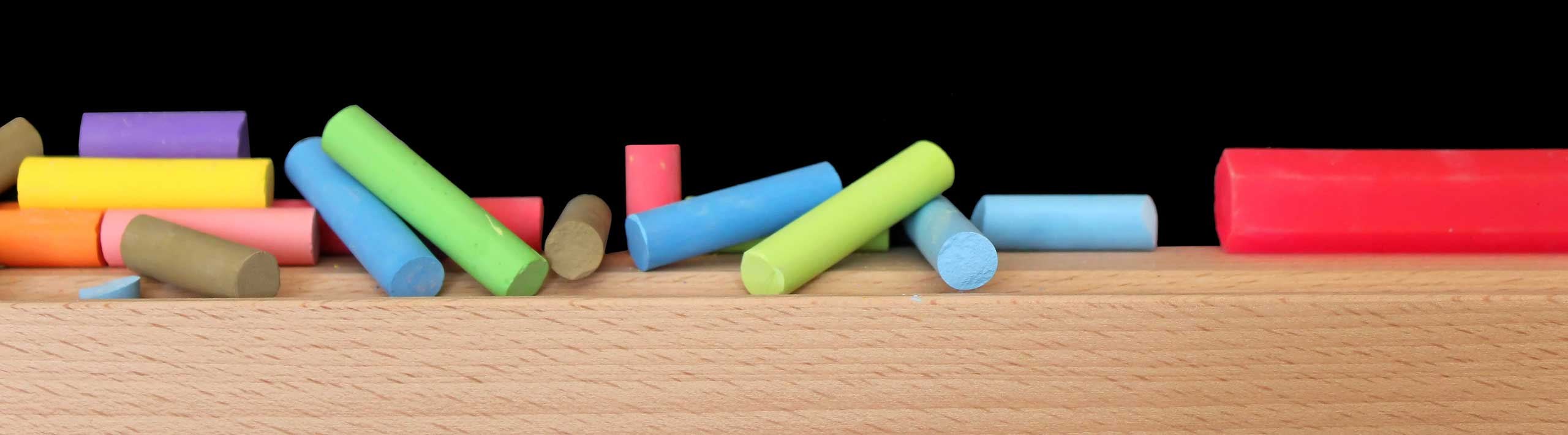# Permutations Calculator

An online calculator to calculate the number of permutations of n elements taken r a the time written as n P r and given by the formula
n P r = P(n , r) = n! / (n-r)!

## Use the Permutations Calculator

1 - Enter the positive integers n and r and press "Calculate Permutations".

 P(2 , 2)

More Math Calculators and Solvers.

and also

Power Calculator.